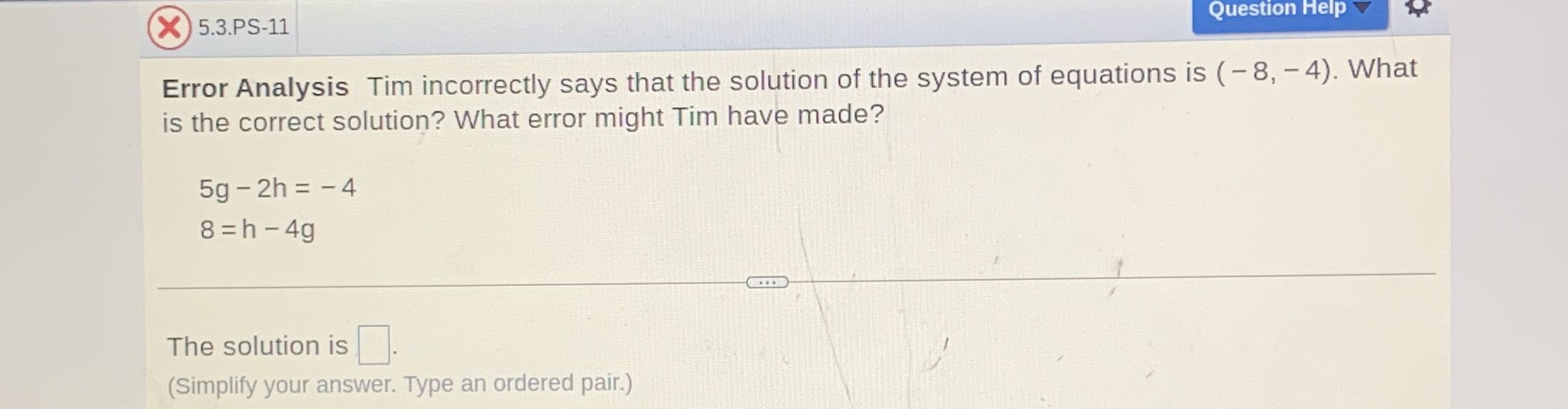### Still have math questions?

Algebra
QuestionTim incorrectly says that the solution of the system of equations is $$( - 8 , - 4 )$$ . What is the correct solution? What error might Tim have made?

$$5 g - 2 h = - 4$$

$$8 = h - 4 g$$

The solution is $$\square$$ . (Simplify your answer. Type an ordered pair.)

$$g= -4,\space h= -8$$